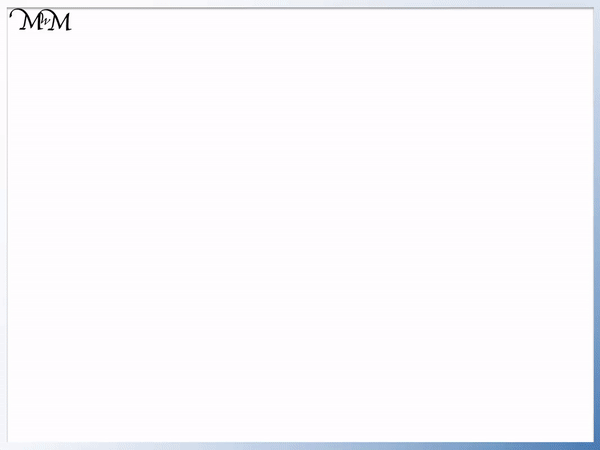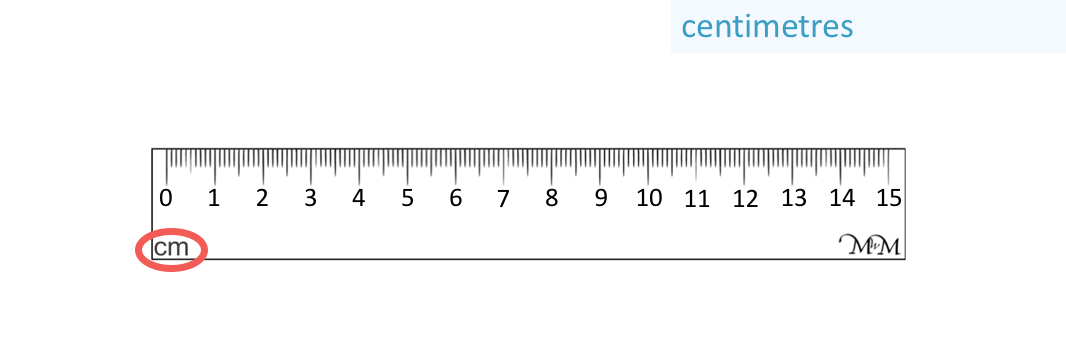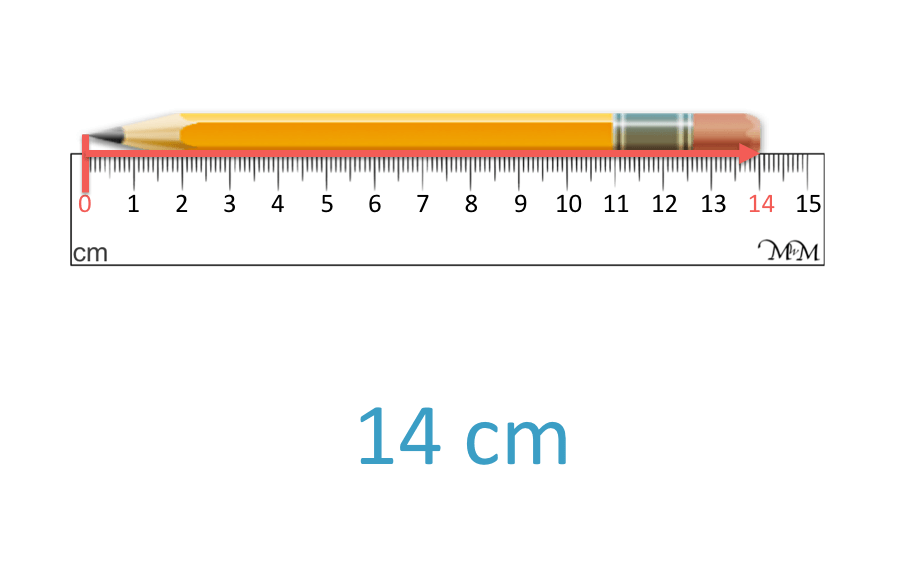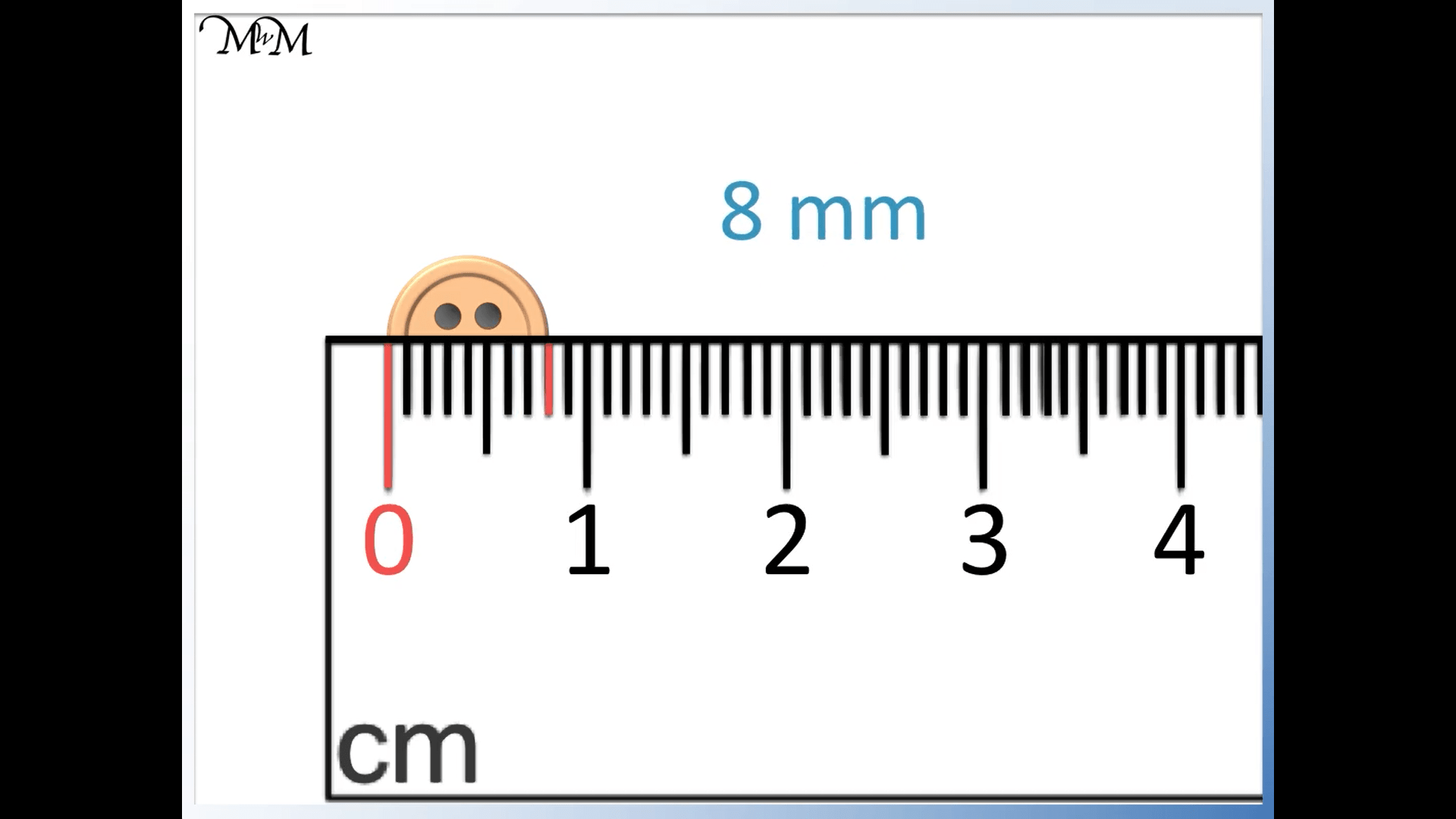# Measuring Centimetres Using a Ruler

Measuring Centimetres Using a Ruler• We can measure how long an object is in centimetres.
• We can write centimetres as ‘cm’ for short.
• Here is a 15 centimetre ruler with ‘cm’ marked on it.
• The distance from each number to the next is one centimetre.
• To measure an item in centimetres, begin by lining it up with zero on the ruler.
We measure objects in centimetres, written as ‘cm’ for short.

The distance between each number on a ruler is one centimetre.• We will measure the length of this eraser in centimetres using a ruler.
• We line the left edge of the eraser up with the line that points to zero on our ruler.
• We look at the right edge of the eraser and it lines up with the 4 on our ruler.
• The length of the eraser is 4 cm.# How do you Measure the Length of an Object in Centimetres Using a Ruler?

Centimetres are units of measurement that we commonly see on rulers and are written with the abbreviation ‘cm’.

Centimetres help to describe how long an object is.

Below is a 15 centimetre ruler. It has numbers from 0 to 15 and each number is a centimetre apart from the number next to it.Centimetres are one of the common metric units of measurement and they are easy to compare to millimetres, metres and kilometres when we describe how long a distance is.

All of these words end in ‘metres’ so we know that we can compare them.

Some rulers have ‘inches’ on the other side and we cannot compare centimetres to inches as easily.

We typically write centimetres as ‘cm’ for short, with a space between the number and the ‘cm’.

The ruler itself is slightly longer than 15 cm, however the distance from the 0 line to the 15 line is 15 cm.

It is important to measure objects using the measurement lines on the ruler.

We always start from the ‘0’ line on the ruler and we line our objects up with this point.A common mistake when learning to measure objects with a ruler is to not line up the object with the zero correctly.

When teaching measuring with a ruler it is important to stress that the measurement is taken from the zero mark and not the edge of the ruler.

Here is our first example of measuring centimetres using a ruler.

We will measure the length of this pencil sharpener.

Remember to line up the left edge of the pencil sharpener with the zero line.The pencil sharpener’s right edge lines up with the 1. This means that the pencil sharper is 1 cm across in width.

Here is another example of measuring centimetres with a ruler.

We line up the left of the eraser with the zero line on the ruler.The right hand side of the eraser lines up with the 4 on the ruler and so, the length of the eraser is 4 cm.

The final example of measuring centimetres using a ruler is given below.

We will measure the length of this pencil by lining its left tip up with the zero mark.The right most tip of the pencil lines up with the 14 line and so, the pencil is 14 cm long.Now try our lesson on Measuring Millimetres on a Ruler where we learn how to measure an object more precisely in millimetres.